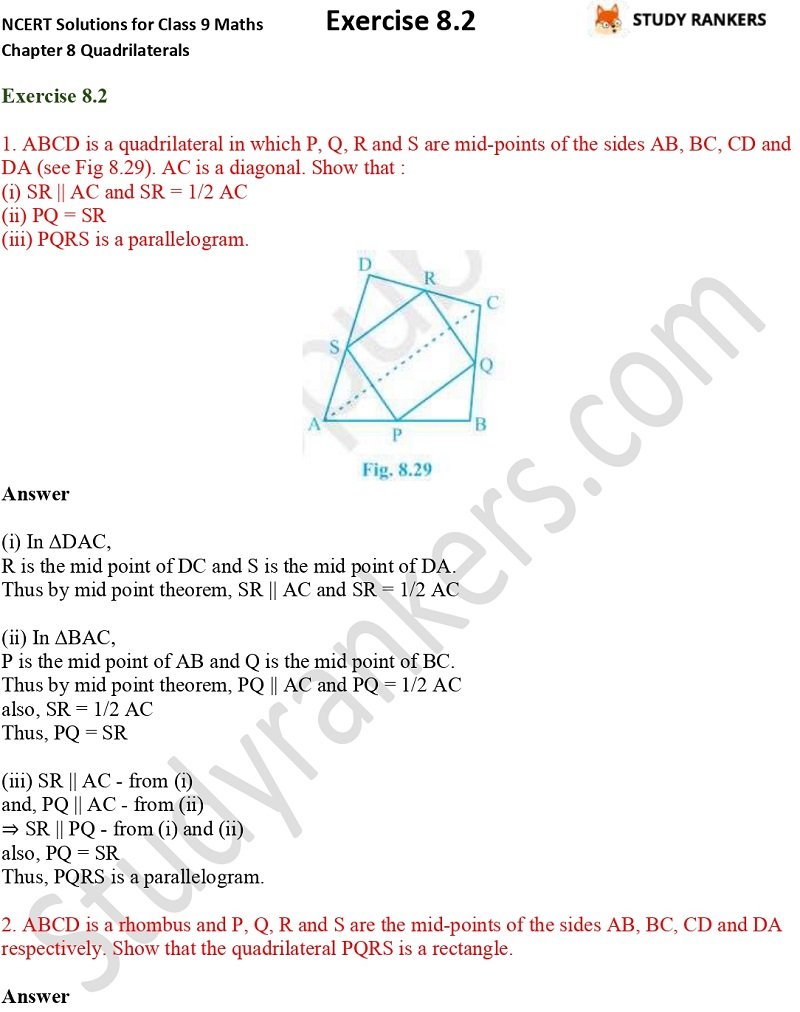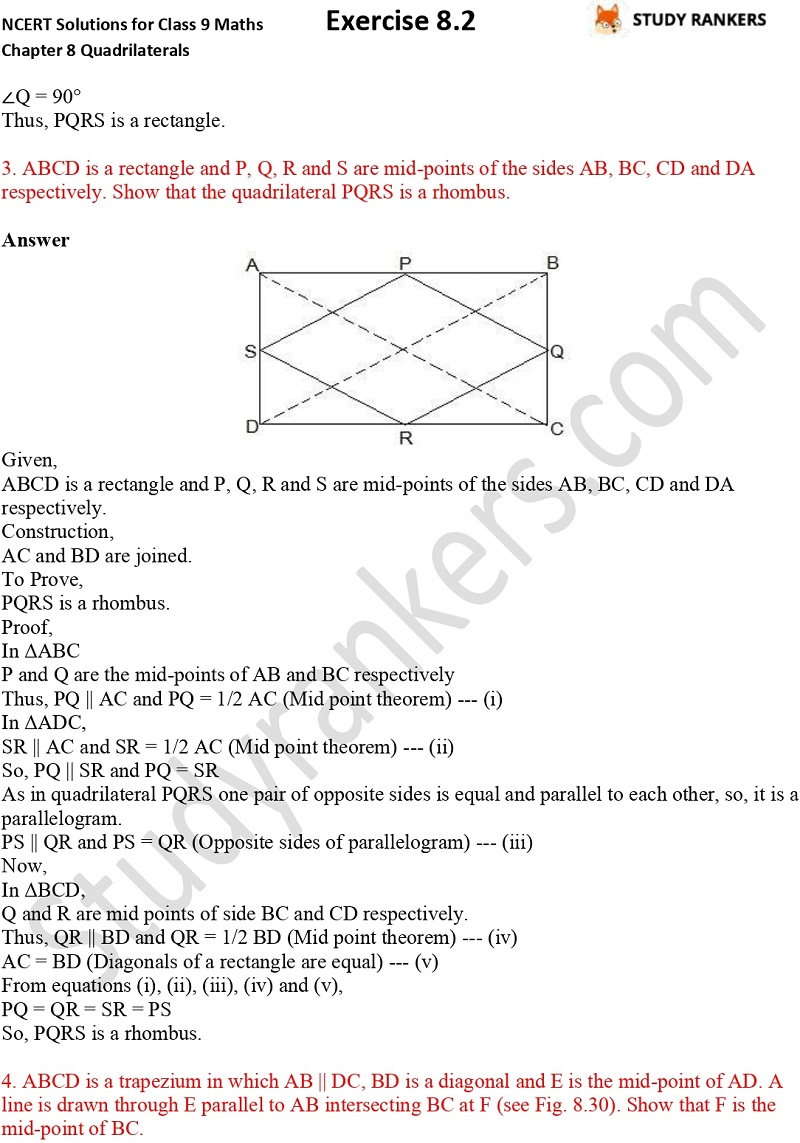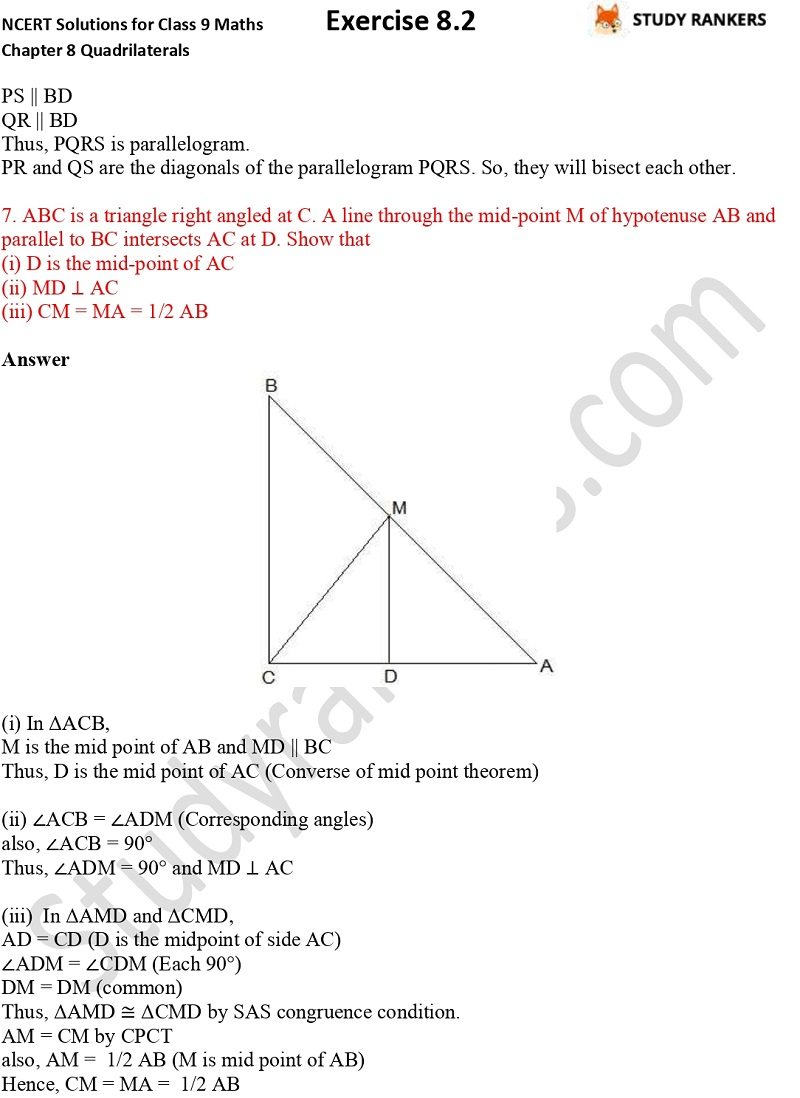>

## NCERT Solutions for Class 9 Maths Chapter 8 Quadrilaterals Exercise 8.2

Chapter 8 Quadrilaterals Exercise 8.2 NCERT Solutions for Class 9 Maths is given here so you can learn the basic concepts of the chapter easily and complete your homework. Class 9 Maths NCERT Solutions provided here will yield better results in the examinations. These NCERT questions and answers are updated according to the latest pattern of CBSE so you don't have to waste precious in finding accurate solutions.

Ex 8.2 has seven questions which are about showing a given statement in the figures.X